Math Concepts
What are the different lines in Math?
68.5k views

 1 What is a Line? 2 Types of Lines 3 Other Types of Lines 4 Conclusion 5 About Cuemath 6 Frequently Asked Questions (FAQs) 7 External References

January 25, 2021

Lines are everywhere around us in our daily life and they are part of our life. This blog helps kids to learn the different types of lines in our life and how and where they are used. Go ahead to explore.

## What is a Line?

A line has length but no width. A line is a type of geometric figure that can move in both directions. A line is made up of an endless number of points. It is infinite and has no ends on both sides. A line is one dimentional.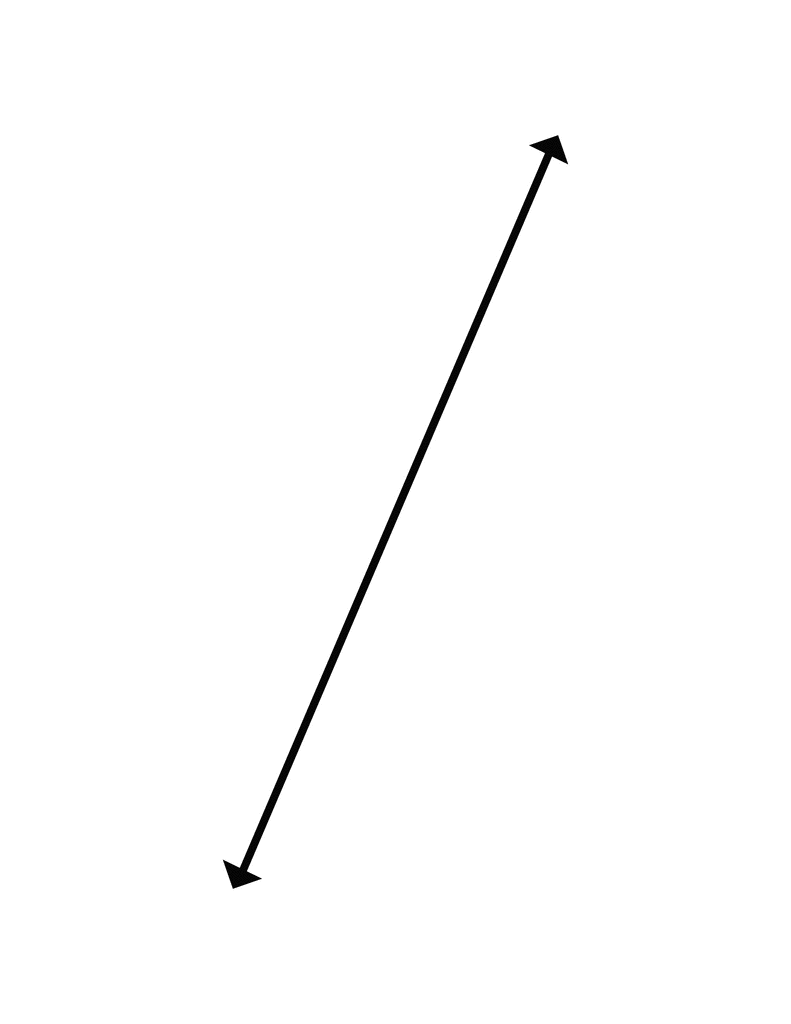In geometry, the notion of line or straight line was introduced by ancient mathematicians to represent straight objects having negligible width and depth. It is often described in terms of two points.

A line in the plane is often defined as the set of points whose coordinates satisfy a given linear equation in the concept of analytic geometry whereas, a line may be an independent object, distinct from the set of points which lie on it in the concept of incidence geometry.

If you ever want to read it again as many times as you want, here is a downloadable PDF to explore more.

## Types of Lines

There are different types of lines used in Geometry. Lines are the foundation of the Geometry. Basically, there are two types of lines:

1. Straight line                                                                                       2. Curved line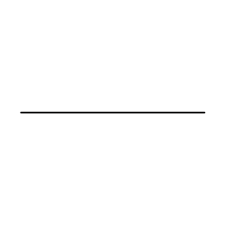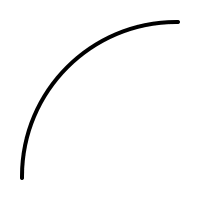Straight lines are further classified into Horizontal lines (Sleeping lines), Vertical lines (Sleeping lines) and Oblique lines (Slanting lines). The equation of straight line is  $$ax+b=0$$.

1. ### Horizontal line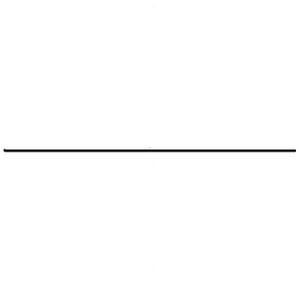Definition: A line that is parallel to x-axis is called as horizontal line. A horizontal line proceeds parallel to the x-axis. This line does not touch any point on the X-axis.
Examples of horizontal lines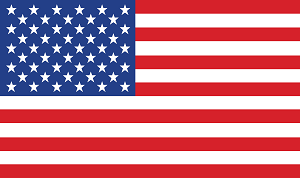The red and white stripes in the flag are one of the examples for horizontal lines. Other popular examples include steps on the staircase, planks on the railway tracks.

1. ### Vertical lineDefinition: A line that is parallel to y -axis is called as vertical line. It  goes straight up and down, parallel to the y-axis of the coordinate plane.

#### Examples of vertical lines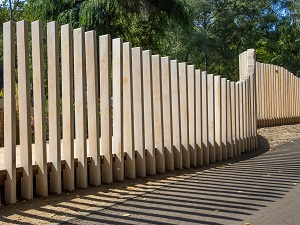The steel rails in a fence are an example of vertical lines. Even, a row of tall trees on a highway, Electric poles placed on the roads are examples of vertical lines.

## Other Types of Lines

When two or more lines are used together, they form different types of lines like parallel lines, perpendicular lines, and transversal lines as shown below.

1. ### Parallel lines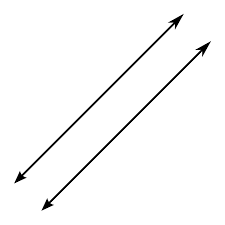When two lines that run together separated by the same distance and do not meet are known as parallel lines, irrespective of the length of the lines. Curves that do not touch each other or intersect and keep a fixed minimum distance are said to be parallel.  Two straight lines in a plane that do not intersect at any point are said to be parallel.

The symbol || is used to represent parallel lines.

#### Real-Life Examples of Parallel LinesRailway tracks are the best example to show the parallel lines.

Walls of buildings, Stack of identical notebooks, collection of same-sized papers are all parallel to each item in the collection when arranged uniformly.

1. ### Intersecting lines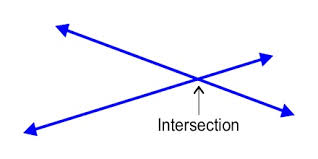Two non-parallel lines may meet at a point and those lines are called intersecting lines. Intersecting lines are two lines that share exactly one point. This shared point is called the point of intersection.

#### Real-Life Examples of Intersecting Lines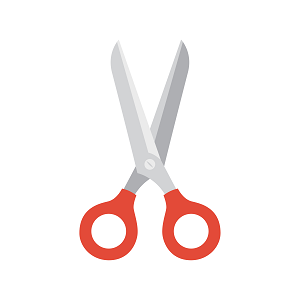Scissors, the two blades of the scissors intersect at each other to make it work effectively.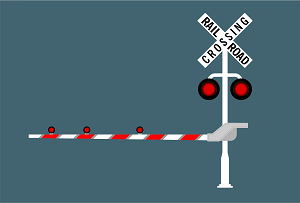These signboards on the roads are one of the best examples to show the intersection of lines.

1. ### Perpendicular lines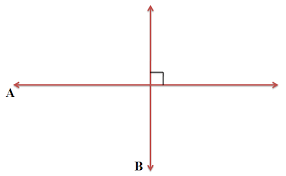A line is said to be perpendicular to another line if the two lines intersect at a right angle. Perpendicular lines are denoted by the symbol of (⊥). This property of being perpendicular is the relationship between two lines which meet at a right angle (90 degrees).

#### Real-Life Examples of Perpendicular LinesClocks are the best examples to show perpendicular lines at 3 O clock and 9 O clock.

##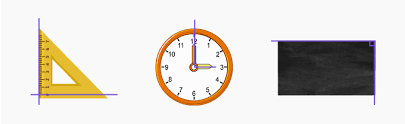Set squares, blackboards are also one of the real-life examples of perpendicular lines. Television sets, bookshelves, and on the globe, where lines of latitude and longitude intersect are all examples of perpendicular lines.

1. ### Transversal line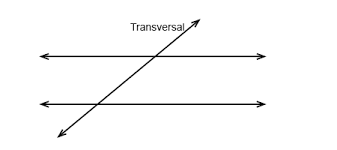The Transversal line is a straight line that cuts two or more lines which may or may not be parallel. A transversal line passes through two lines in the same plane at two distinct points in the concept of geometry. Transversals play a role in establishing whether two other lines in the Euclidean plane are parallel.

##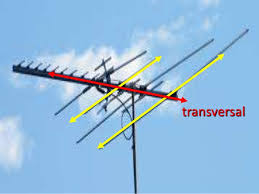These are some of the examples of the transversal lines in our daily life, the antenna, stair railings.

Apart from these, there are few more types of lines, about which the kids can explore such as skew lines, coplanar lines, concurrent lines, etc.

## Conclusion

So, the line can be defined as a straight one-dimensional figure that has no thickness and extends endlessly in both directions.

Lines are one of the important aspects and introduction to Geometry. With the types of lines introduced in this blog, kids will be able to apply their ideas to real life and explore their thoughts.

Cuemath, a student-friendly mathematics and coding platform, conducts regular Online Classes for academics and skill-development, and their Mental Math App, on both iOS and Android, is a one-stop solution for kids to develop multiple skills.Understand the Cuemath Fee structure and sign up for a free trial.